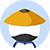If N is a positive integer, does N have exactly three distinct positive factors?

Statement #1: the integer $N^2$has exactly five distinct factors

Statement #2: only one factor of N is a prime number
• AStatement 1 ALONE is sufficient to answer the question, but statement 2 alone is NOT sufficient.
• BStatement 2 ALONE is sufficient to answer the question, but statement 1 alone is NOT sufficient.
• CBOTH statements 1 and 2 TOGETHER are sufficient to answer the question, but NEITHER statement ALONE is sufficient.
• DEach statement ALONE is sufficient to answer the question.
• EStatement 1 and 2 TOGETHER are NOT sufficient to answer the question. Previous

• 网友解析

### 题目讨论

• 优质讨论
• 最新讨论• 所属科目:数学DS
• 题目来源1:Magoosh-212
• 正确率: 58%
• 难度: